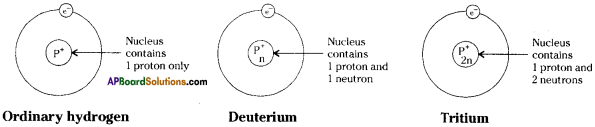AP State Syllabus AP Board 9th Class Physical Science Important Questions Chapter 11 Sound

## AP State Syllabus 9th Class Physical Science Important Questions 11th Lesson Sound

### 9th Class Physical Science 11th Lesson Sound 1 Mark Important Questions and Answers

Question 1.
Mention two important devices used in SONAR system?
Sonar system consists of a transmitter and a detector.

Question 2.
A sound of wavelength 0.6 cm is travelling in air with a velocity of 300 m/s. Is this sound audible?
We know that v = nλ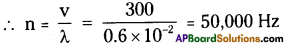This sound is not in the audible range. Hence this is not audible.Question 3.
How do we get the sensation of sound?
Sound travels in air due to the to and fro motion of the air particles, which act upon the ear and produce the sensation of sound.

Question 4.
On which factor does the pitch of a tuning fork depend?
The pitch of the tuning fork depends on the length of the prongs.

Question 5.
How does the sound travel?
Sound travels^in the form of waves.

Question 6.
What are longitudinal waves?
If the particles of the medium vibrate along the direction of wave, the wave is called a longitudinal wave.Question 7.
Which wave involves change in the density of the medium?
Longitudinal wave involves change in the density of the medium.

Question 8.
What do you call the regions formed in longitudinal wave propagation?
The regions formed during the longitudinal wave propagation due to change in density are compressions and rarefactions.

Question 9.
When do we call a wave as transverse wave?
If the particles of the medium vibrate perpendicular to the direction of wave, then the wave is called a transverse wave.

Question 10.
What is an echo?
A reflection of sound, arriving at the listener in more than 0.1 sec after direct sound is called an echo.Question 11.
What is reverberation?
A reflection of sound, arriving at the listener, in less than 0.1 sec after direct sound is called reverberation.

Question 12.
What is the audible range of an ordinary person?
20 Hz to 20 K.Hz.

Question 13.
Give examples to the animals which produce infrasonics.
Animals such as elephants and whales produce infrasonics.

Question 14.
Expand SONAR.
SONAR stands for Sonographic Navigation and Ranging.

Question 15.
Define a compression and a rarefaction.

1. Compressions are the regions where density, as well as pressure of particles, is high.
2. Rarefactions are the regions where the density, as well as pressure of particles, is low.

Question 16.
Define time period of the sound wave.
The time taken to complete one oscillation of the density of the medium is called the time period of the sound wave, denoted by T, and unit in S.I. system is second.

Question 17.
Define speed of a sound wave.
The distance by which a point on the wave, such as a compression or rarefaction, travels in unit time is called speed of sound wave.Question 18.
In which of the three media air, water, or iron sound travel the faster at a particular temperature?
Sound travels faster in iron because speed of sound is maximum in solids then liquids and least in gases.

Question 19.
What is audiable range of the average human ear?
The audiable range of the average human ear is 20 Hz to 20 KHz.

Question 20.
Flash and thunder are produced simultaneously. But thunder is heard a few seconds after the flash is seen why?
Speed of sound is less (i.e., 342 m/s) is less than speed of light (i.e., 3 x 108 m/s). So, thunder is heard a few seconds after the flash is seen.

Question 21.
The frequency of source of sound is 100 Hz. How many times does it vibrates in 1 minute?
Frequency (υ) = 100 Hz = 100 vibrations/ second.
Number of vibrations in minute = 100 × 60 = 6000 vibrations/ minute.

Question 22.
Which fundamental particles were discovered by
i) Thomson
ii) Goldstein
i) Thomson – Electron
ii) Goldstein – Proton

Question 23.
What is a nucleus?
The small positively charged central part of an atom is called nucleus.Question 24.
What is an orbit?
Orbit is the path of the electron around the nucleus.

Question 25.
What will the addition of a neutron to the nucleus of an atom do?
It will increase the atomic mass of the atom.

Question 26.
Which constituent particles of the atom determine the following
a) size of the atom
b) change on the nucleus?
a) Electrons.
b) Protons.

Question 27.
Magnesium atoms has 12 electrons. Which energy shell is incomplete?
M – shell is incomplete.

Question 28.
If K and L shells of an atom are full, then what would be the total number of electrons in the atom?
K – 2 ; L – 8.
2 + 8 = 10 is the total number of electrons if K and L – shells are full.

Question 29.
An atom of an element has 7 electrons in its L shell
a) What is its atomic number?
b) State its valency.
a) Atomic number = 9 [∴ K : 2 ; L : 7]
b) Valency is ‘I’.

Question 30.
The atomic number and mass number of an element are 11 and 23 respectively. Find the number of neutrons in its nucleus.
Number of neutrons (N) = Mass number (A) – Atomic number (Z) = 23 – 11 = 12.

Question 31.
The mass number of chlorine atom is 35 and its atomic number is 17. How will this chlorine atom be represented?
$${ }_{17}^{35} \mathrm{Cl}$$

Question 32.
What is an anion?
When an atom gains one or more electrons, it becomes negatively charged and is known as anion.Question 33.
Give one example each of diatomie and triatomic molecules.

1. Diatomic molecule – Oxygen (O2)
2. Triatomic molecule – Ozone (O3)

Question 34.
If Z = 3, what would be the valency of the element?

1. If Z = 3, the distribution of electrons is (2,1).
2. Thus the valency of the Element is 1.

Question 35.
Fluorine atom has 9 electrons and 9 protons. How many energy shells it has?
Two energy shells. [∴ K = 2 ; L = V]

Question 36.
Which subatomic particle is not present in an ordinary hydrogen atom?
Neutron.

Question 37.
What is the maximum number of electrons which can be accommodated in the
a) innermost shell of an atom?
b) outermost shell of an atom?
a) 2
b) 8

Question 38.
What is the usual symbol for a) an electron b) a proton and c) a neutron?
a) electron = e
b) proton = p+
c) neutron = n°

Question 39.
Name the shell of an atom which can accommodate a niaximum of a) 8 electrons b) 18 electrons.
a) L-shell

Question 40.
Name the negatively charged particle present in the atones of all the elements.
electron (e).

Question 41.
Which part of an atom was discovered by Ruthorford’s alpha particle scattering experiment?
Nucleus.Question 42.
State the relative mass and charge of a proton.

1. Relative mass = 14
2. Relative charge = + 1

Question 43.
Name the radioactive isotope which is used in the treatment of cancer.
Cobalt – 60.

Question 44.
Which radioactive isotope is used to determine the activity of thyroid gland?

Question 45.
What name is given to the pair of atoms such as $${ }_{7}^{14} \mathrm{N}$$ and $${ }_{7}^{15} \mathrm{N}$$?
Isotopes.

Question 46.
Which noble gas has less than 8 electrons in the valence shell of its atom?
Helium.Question 47.
What is the general name of the elements having 8 electrons in the valence of shell of their atoms?
Noble gases.

### 9th Class Physical Science 11th Lesson Sound 2 Marks Important Questions and Answers

Question 1.
How can you say that the sound waves are longitudinal?

1. When sound wave passes through air, the layers in the medium are alternately pushed and pulled.
2. Thus the particles of the medium move to and fro along the direction of propagation.
3. Therefore sound waves in air are longitudinal.

Question 2.
What are the characteristics of a sound wave?
The characteristics of a sound wave, which play an important role in describing the nature of a wave are

1. Wavelength (λ)
2. Amplitude (A)
3. Frequency (υ)
4. Wave speed (v)

Question 3.
Deduce a relationship between time period and frequency of a sound wave.

1. Let the time taken for o oscillations = 1 sec.
2. The time taken for one oscillation = $$\frac{1}{υ}$$ sec.
3. But the time taken for one oscillation is called the time period (T) and the number of oscillations per second is called the frequency (υ).
4. Hence frequency and time period are related as T = $$\frac{1}{υ}$$ (or) υ = $$\frac{1}{T}$$Question 4.
What is the speed of sound wave in air, water and iron at 20 °C?
The speed of sound in air at 20°C is 343.2 m/s.
The speed of sound in water at 20°C is 1484 m/s.
The speed of sound in iron at 20°C is 5120 m/s.

Question 5.
Define echo and reverberation.
Echo :
A reflected sound arriving at the position of listener in more than 0.1 s after the direct sound is called an echo’.

Reverberation :
A reflection of sound, arriving at the listener in less than 0.1 s after the direct sound is called reverberation’.

Question 6.
Explain the working of a megaphone and a horn.
(Or)
How multiple reflections of sound are used in working of mega phone and a horn?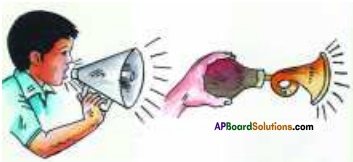In megaphone and a horn, a tube followed by a conical opening reflects sound successively to guide most of the sound waves from the source in the forward direction towards the audience.

Question 7.
Describe the working of a stethoscope.1. Stethoscope is a medical instrument used for listening to sounds produced with in the body, chiefly in heart or lungs.
2. In stethoscopes the sound of the patient’s heartbeat reaches the doctor’s ears by multiple reflection and amplifying the sound.

Question 8.
How the concert hails and cinema halls are designed to use multiple reflections of sound?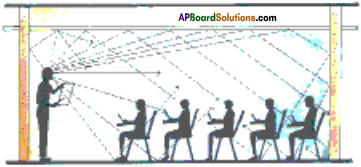Generally the ceilings of concert halls, conference halls and cinema halls are designed such that the sound after reflection reaches all corners of the hall as shown in the figure.

In some halls, a curved ceiling is arranged in such a way that the sound after reflecting from the ceiling spreads evenly across the hall.

Question 9.
What are the medical applications of ultrasound?
1) Imaging of organs :
Ultrasonics are useful in Electro Cardio Graphy (ECG) to form an image of heart.

Ultrasonography is used in the formation of images of organs such as liver, gall bladder, uterus, etc. to identify the abnormalities and tumors. Ultrasonography is also used to monitor the growth of a foetus inside the mother’s womb.

2) Surgical use of ultrasound :
Ultrasounds are used in cataract removal, breaking up of stones in kidneys, etc. without surgery.

Question 10.
Show the frequencies of various musical notes in a table.
In musical terms, the pitch of the note determines the position of the note on the musical scale which is denoted as :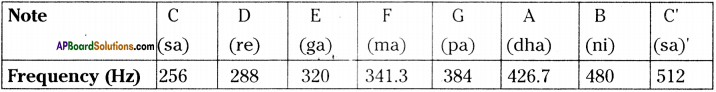The tuning fork set is prepared based on the above frequencies.

Question 11.
Draw a diagram to show wavelength and amplitude of a wave.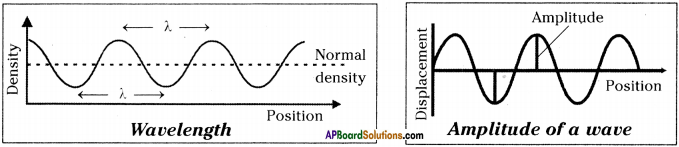Question 12.
Calculate the wavelength of a sound wave whose frequency is 220 Hz and speed is 440 m/s in a given medium.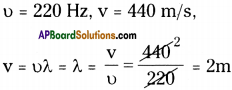Question 13.
An echo returned in 3s. What is the distance of the reflecting surface from the source, given that the speed of sound is 342 m/s?
Speed of sound (v) = 342 m/s, Time taken to heard echo, t = 3 s.
Distance travelled by sound = v × t = 342 × 3 = 1026 m.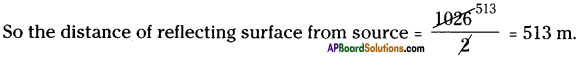Question 14.
What are the range of frequencies associated with (a) infrasonic sound, b) ultrasonic sound?
a) For infrasonic sound frequency is less than 20 Hz.
b) For ultrasonic sound frequency is greater than 20 KHz.Question 15.
Which characteristic of sound helps you to identify your friend by his voice while sitting with others in a dark room?
The pitch of voice differs for person to person which inturn depends on frequency. So, the frequency is the characteristic of sound which helps to identify my friends voice.

Question 16.
A person has hearing range from 20 Hz to 20 KHz. What are the typical wavelength of sound waves in air corresponds to these two frequencies? Take speed of sound in air as 344 m/s.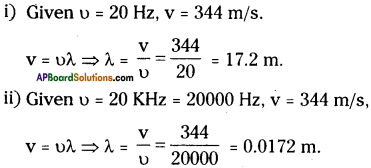Question 17.
A sound wave travels at a speed of 339 m/s. If its wavelength is 1.5 cm. What is the frequency of the wave? Will it be audiable?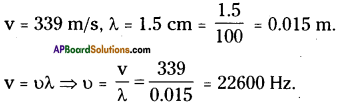It is more than 20000 Hz (20 KHz). So the sound is not audiable.

Question 18.
A sonar device on a submarine sends out a signal and receives an echo 5 s later. Calculate the speed of sound if the distance of an object from the submarine is 3625 m.
Distance of an object from the submarine (d) = 3625 m.
The time taken to receive signal (t) = 5s.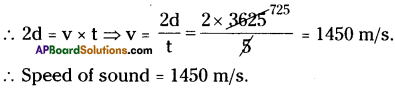Question 19.
Fill in the following blanks in respect of an atom of an element.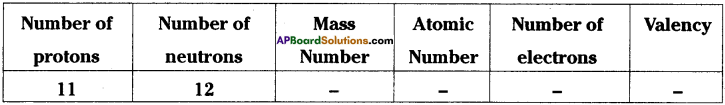Mass number = 23
Atomic number =11
Number of electrons =11
Valency = 1

Question 20.
Write the electronic configurations of the following elements.
a) Carbon
b) Neon
c) Chlorine
d) Calcium
a) 2, 4
b) 2, 8
c) 2, 8, 7
d) 2, 8, 8, 2

Question 21.
Helium atom has an atomic mass of 4u and two protons in its nucleus. How many neutrons does it have?
1. Mass number = No. of protons + No. of neutrons
⇒ 4 = 2 + No. of neutrons
⇒ No. of neutrons = 4 – 2 = 2
2. Thus, the helium atom has 2 neutrons.

Question 22.
Fill in the following blanks.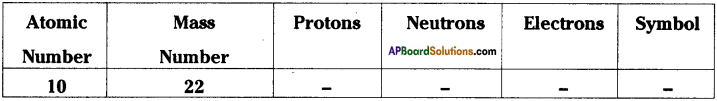1. Protons : 10
2. Neutrons : 12
3. Electrons : 10
4. Symbol : Ne

Question 23.
Write the three isotopes of carbon and hydrogen.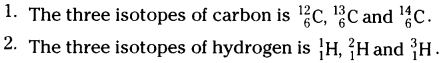Question 24.
Three different atoms of oxygen are represented as $${ }_{8}^{16} O,{ }_{8}^{17} O, \text { and }{ }_{8}^{18} O$$.
a) What do the lower figures and upper figures represent ?
b) Give the nuclear composition of $${ }_{8}^{18} \mathrm{O}$$.
a) Lower figures → Atomic number
Upper figures → Mass number

b) Composition of $${ }_{8}^{18} \mathrm{O}$$ → Protons : 8
Neutrons : 18 – 8 = 10Question 25.
An atom of an element has 6 electrons in L shell.
a) What is the atomic number of the element?
b) State its valency.
c) Identify the element and write its name. [ K = 2 ; L = 6 Given]
a) Atomic number = 2 + 6 = 8
b) Valency = 2
c) Oxygen atom.

Question 26.
State any two similar properties of isotopes.

1. Isotopes of an element have same atomic number.
2. Isotopes of an element have similar number of electrons.

Question 27.
If chlorine atom is available in the form of Say, two isotopes $${ }_{17}^{35} \mathrm{Cl}$$ (75%) and $${ }_{17}^{35} \mathrm{Cl}$$ (25%), calculate the average atomic mass of chlorine atom.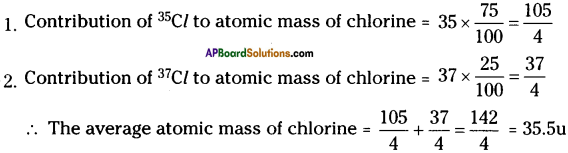Question 28.
Write the name of any two radioactive isotopes.
1. Uranium – 235
2. Cobalt – 60

### 9th Class Physical Science 11th Lesson Sound 4 Marks Important Questions and Answers

Question 1.
“Sound travels in the form of waves”. Justify your argument.

1. Sound is a form of energy which travels through the air and reaches our ears to give the sensation of sound.
2. There may be two possible ways by which transfer of energy from the source of sound to our ears take place.
a) Source of sound produces disturbances in air and they strike our ears.
b) Some particles are shot off from the source of sound and they reach our ears.
3. If the second explanation is correct, the vibrating body would gradually lose its weight as particles are continuously shot off from it.
4. This is impossible because it would lead to vanishing of the object.
5. Hence the first explanation that the sound travels through disturbances in the form of waves is correct.

Question 2.
Mention the industrial applications of ultrasonic waves.
1) Drilling holes and making cuts of desired shapes :
a) Holes can also be drilled using ultrasonic vibrations.
b) Ultrasonic cutting and drilling are very effective for fragile materials like glass, etc.

2) Ultrasonic cleaning :
a) Ultrasonics help in cleaning the parts located in hard-to-reach places.
b) The high frequency ultrasonic vibrations knocks off all dirt and grease particles from the objects.

3) Ultrasonic detection of defects in metals :
The defects in the metallic structures, which are not visible from the outside, can be detected by ultrasonic waves.Question 3.
Draw the graphical representation of
a) Lower pitch, higher pitch
b) Louder sound, soft sound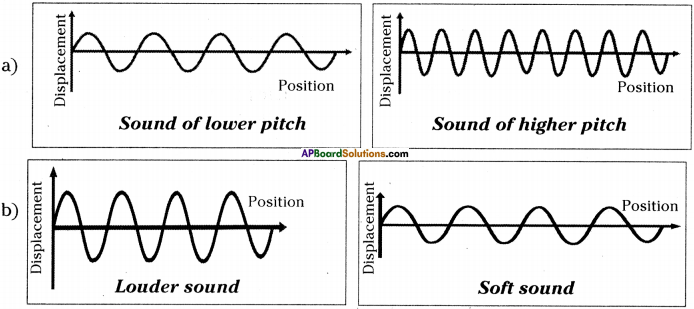Question 4.
Draw the graphical representation of sound wave produced by a tuning fork, a violin and a piano playing the same note with equal loudness.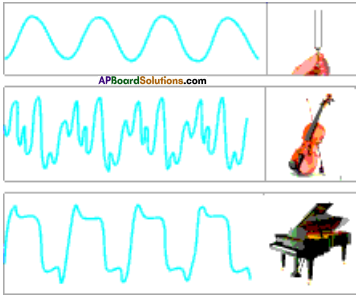Question 5.
Composition of the nuclei of two atomic species X and Y are given as under.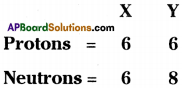a) Give the mass number of X and Y.
b) What is the relation between the two species ?
a) Mass number of X = 6 + 6 = 12
Mass number of Y = 6 + 8 = 14

b) Since X and Y both have atomic numbers as 6 but mass numbers are different.
∴ These are isotopes to each other.

Question 6.
How will you find the valency of Magnesium, Sulphur, and Chlorine?
Electronic configuration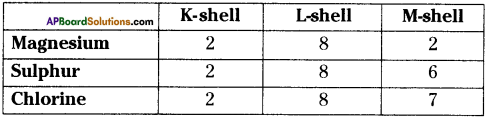1. Valency of Magnesium = 2
2. Valency of Sulphur = 8 – 6 = 2
3. Valency of Chlorine = 8 – 7 = 1

Question 7.
For the symbol H, D and T tabulate three sub-atomic particles found in each of them.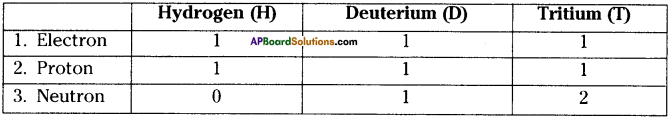Question 8.
Draw a sketch of Bohr’s model of an atom with three shells.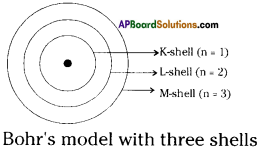Question 9.
Observe the following table and answer the following questions.

 X Y Electrons 8 8 Protons 8 8 Neutrons 8 9

a) What is the mass number of X?
b) What is the mass number of Y?
c) What is the relation between X and Y?
d) Which element do they represent?
a) Mass number of X = 16 (∵ number protons + number neutrons)
b) Mass number of Y = 17
c) Isotopes
d) Oxygen (168O)

Question 10.
Draw the diagrams of the three isotopes of hydrogen and label the parts.